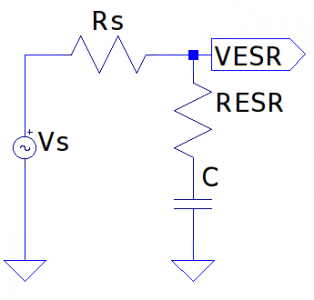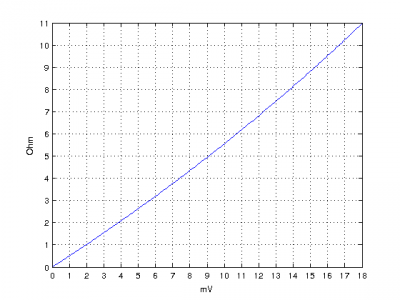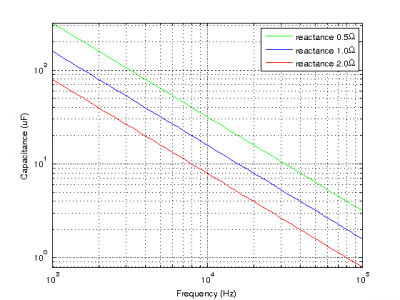Capacitor ESR (Equivalent Series Resistance) measurement is very useful in diagnosing issues with power supplies. In linear power supplies, a high ESR filter capacitor may cause excessive ripple current on the voltage rails and can cause the capacitor to overheat due to its increased resistance. In low dropout linear supplies, the ESR of the output capacitors affect the loop stability and excessive ESR in these output capacitors can make the power supply unstable, which in turn can lead to out-of-tolerance voltage being applied to the load and could cause future damages. In switching mode power supplies, the ESR of capacitors are even more critical. In this blog post I will discuss how to measure the ESR of a capacitor using a function generator and a multimeter. A short video on this topic is also included towards the end.

A typical capacitor can be modeled as an ideal capacitor in series with a resistor–the equivalent series resistance. If we apply an AC voltage to the capacitor under test via current limiting resistor, we have the following circuit:The circuit can be thought as a simple resistor divider if the frequency of the AC source is sufficiently high since the reactance of the capacitor is inversely proportional to the frequency applied for any given capacitance. Thus, we can use the measured voltage across the capacitor to calculate the ESR:

$V_{ESR} \approx V_s \frac{R_{ESR}}{R_{ESR} + R_s}$

Solving for ESR we get:

$R_{ESR} \approx \frac{R_s}{\frac{V_s}{V_{ESR}} – 1}$

If we use a 50 Ohm output impedance function generator to provide the AC input we can hook the capacitor under test directly to the output of the function generator and measure the AC voltage across the capacitor and calculate the ESR using the equation above.

How do we determine what voltage to use? Since electrolytic capacitors are polarized, we can either use an AC voltage with a fixed DC bias or simply use an AC voltage low enough so that the capacitor under test is never subjected to more than its maximum reverse voltage (typically less than 1V). Most of the ESR meters use this second approach as it is easy to implement and we do not need to worry about the polarity of the measurement. Here we choose 100mV as our measurement voltage. This voltage is chosen as it is below P/N junction’s forward voltage (roughly 0.2V to 0.7V depending on type) so that we can perform ESR measure in-circuit.

The graph below plots the calculated capacitor ESR against the measured voltage when an 100mV signal from a 50 Ohm source is used.Our calculation so far is based on the assumption that the reactance of the capacitor is near zero. So in order to get the most accurate result, it is important to choose a measurement frequency based on the capacitor value so that the reactance is ignorable. Recall that the reactance of a capacitor is:

$X_c = -\frac{1}{2\pi f C}$

If we ignore the sign and fix the reactance, we can obtain the relationship of capacitance versus frequency. The following graph shows such relationships for three reactance values (0.5, 1, 2).This graph is useful in determining the minimum frequency needed for the measurement for a given capacitance in order for the reactance to stay below a predetermined value. For instance, if we have a 10 uF capacitor, the minimum frequency needed to ensure that the reactance is under 2 Ohm is roughly 8 kHz. If we want the reactance to be less than 1 Ohm, then the minimum frequency needed is around 16 kHz. And if we want to reduce the reactance further to 0.5 Ohm, we will need to adjust the frequency to above 30 kHz.

While higher frequencies in theory is better for ESR measurement because of the reduced reactance, it is not always desired. This is because the reactance due to the inductance in the circuit increases proportionally as the input frequency increases and this reactance could significantly skew the measurement result. So for large filter capacitors, the frequency used is typically between 1 to 5 kHz and for smaller capacitors a higher frequencies between 10 kHz and 50 kHz can be used. Note that in order to measure the high frequency AC voltage accurately, you will need to use a multimeter with comparable bandwidth (e.g. Keithley 197).